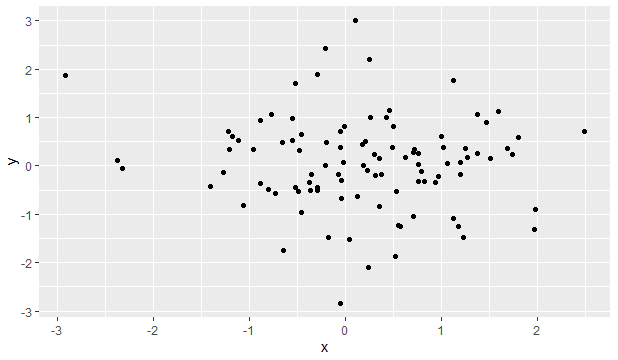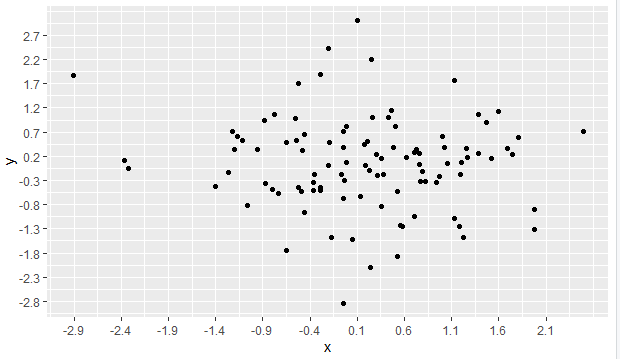2 views

I'm generating plots for some data, but the number of ticks is too small, I need more precision on the reading.

Is there some way to increase the number of axis ticks in ggplot2?

I know I can tell ggplot to use a vector as axis ticks, but what I want is to increase the number of ticks, for all data. In other words, I want the tick number to be calculated from the data.

Possibly ggplot do this internally with some algorithm, but I couldn't find how it does it, to change according to what I want.

by

To increase the number of axis ticks, use the scale_x_continuous and scale_y_continuous functions to override the existing scales.

For example:

For the following plot:To increase the number of ticks:

ggplot(dat, aes(x,y)) +

geom_point() +

scale_x_continuous(breaks = round(seq(min(dat\$x), max(dat\$x), by = 0.5),1)) +

scale_y_continuous(breaks = round(seq(min(dat\$y), max(dat\$y), by = 0.5),1))

Output: